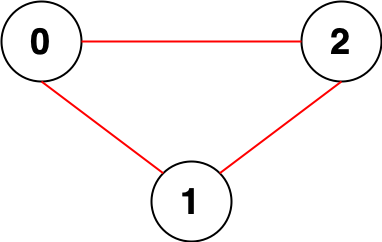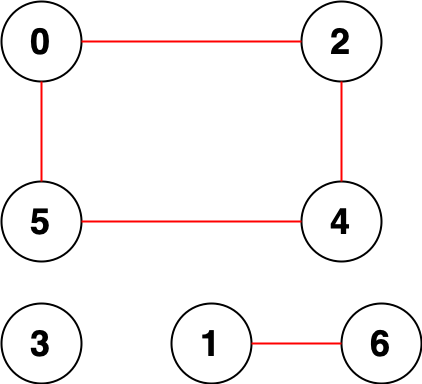2316. Count Unreachable Pairs of Nodes in an Undirected Graph
Medium
1.8K
40

You are given an integer `n`. There is an undirected graph with `n` nodes, numbered from `0` to `n - 1`. You are given a 2D integer array `edges` where `edges[i] = [ai, bi]` denotes that there exists an undirected edge connecting nodes `ai` and `bi`.

Return the number of pairs of different nodes that are unreachable from each other.

Example 1:```Input: n = 3, edges = [[0,1],[0,2],[1,2]]
Output: 0
Explanation: There are no pairs of nodes that are unreachable from each other. Therefore, we return 0.
```

Example 2:```Input: n = 7, edges = [[0,2],[0,5],[2,4],[1,6],[5,4]]
Output: 14
Explanation: There are 14 pairs of nodes that are unreachable from each other:
[[0,1],[0,3],[0,6],[1,2],[1,3],[1,4],[1,5],[2,3],[2,6],[3,4],[3,5],[3,6],[4,6],[5,6]].
Therefore, we return 14.
```

Constraints:

• `1 <= n <= 105`
• `0 <= edges.length <= 2 * 105`
• `edges[i].length == 2`
• `0 <= ai, bi < n`
• `ai != bi`
• There are no repeated edges.
Accepted
73.2K
Submissions
144.8K
Acceptance Rate
50.5%

Seen this question in a real interview before?
1/4
Yes
No

Discussion (0)

Related Topics